# Edgeworth series

2010 Mathematics Subject Classification: Primary: 60F05 [MSN][ZBL]

The series defined by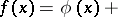(*)Hereis the distribution density of the random variable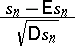(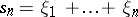, where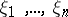are independent and identically distributed),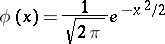is the density of the standard normal distribution, and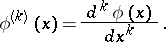The coefficients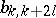,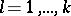, do not depend onand are polynomials with respect to, where,is the variance, and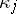is the semi-invariant of orderof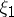. In particular, the first terms of the expansion have the form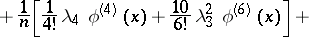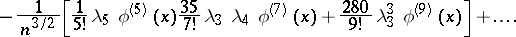The coefficientscan also be expressed in terms of the central moments.

The series (*) were introduced by F.Y. Edgeworth [E]. Their asymptotic properties have been studied by H. Cramér, who has shown that under fairly general conditions the series (*) is the asymptotic expansion ofin which the remainder has the order of the first discarded term.